# Multiplication for Elementary School Chapter Exam

Exam Instructions:

Choose your answers to the questions and click 'Next' to see the next set of questions. You can skip questions if you would like and come back to them later with the yellow "Go To First Skipped Question" button. When you have completed the practice exam, a green submit button will appear. Click it to see your results. Good luck!

Answered 0 of 15

### Page 3

#### Question 11 11. Multiply 34 x 12 by counting intersections. Try to get the answer before the animation completes.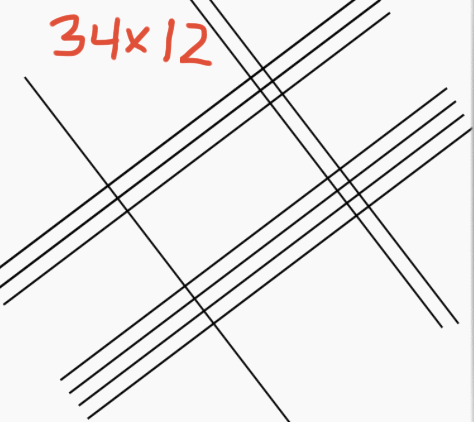#### Question 12 12. Use this diagram to find the number of hundreds, tens, and ones when multiplying 33 by 32.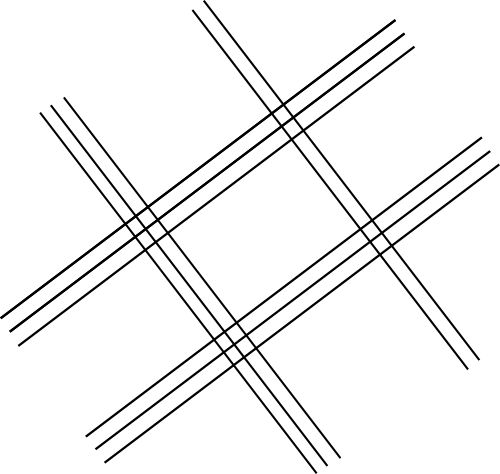#### Question 13 13. What two digit multiplication does this diagram represent?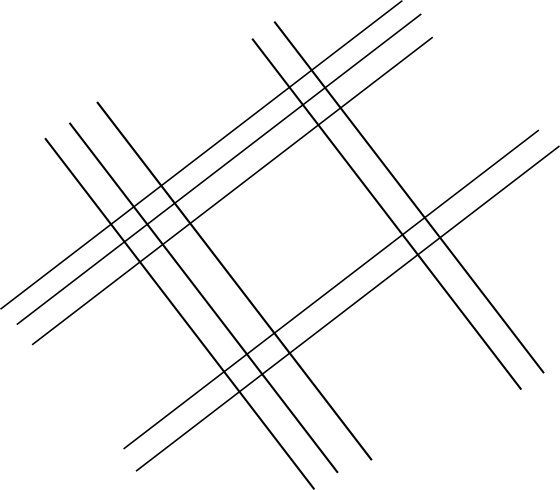#### Question 14 14. Use this diagram to multiply 42 x 56. Count the intersections, and calculate the number of hundreds, tens, and ones. Then choose the correct sum below.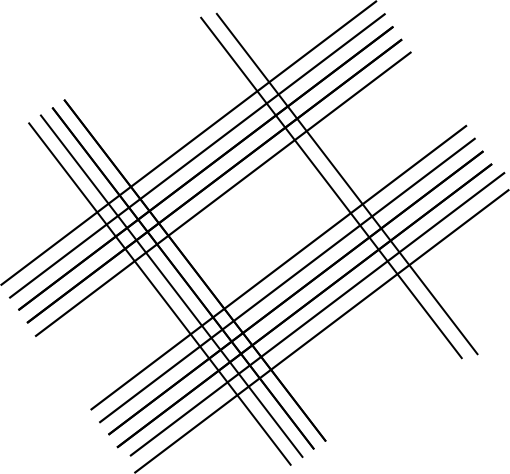#### Question 15 15. Study the visual multiplication problem, and fill in the blank marked with a red question mark.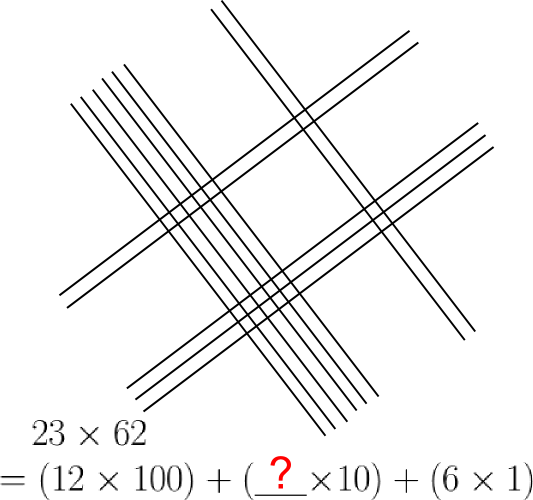#### Multiplication for Elementary School Chapter Exam Instructions

Choose your answers to the questions and click 'Next' to see the next set of questions. You can skip questions if you would like and come back to them later with the yellow "Go To First Skipped Question" button. When you have completed the practice exam, a green submit button will appear. Click it to see your results. Good luck!

Support